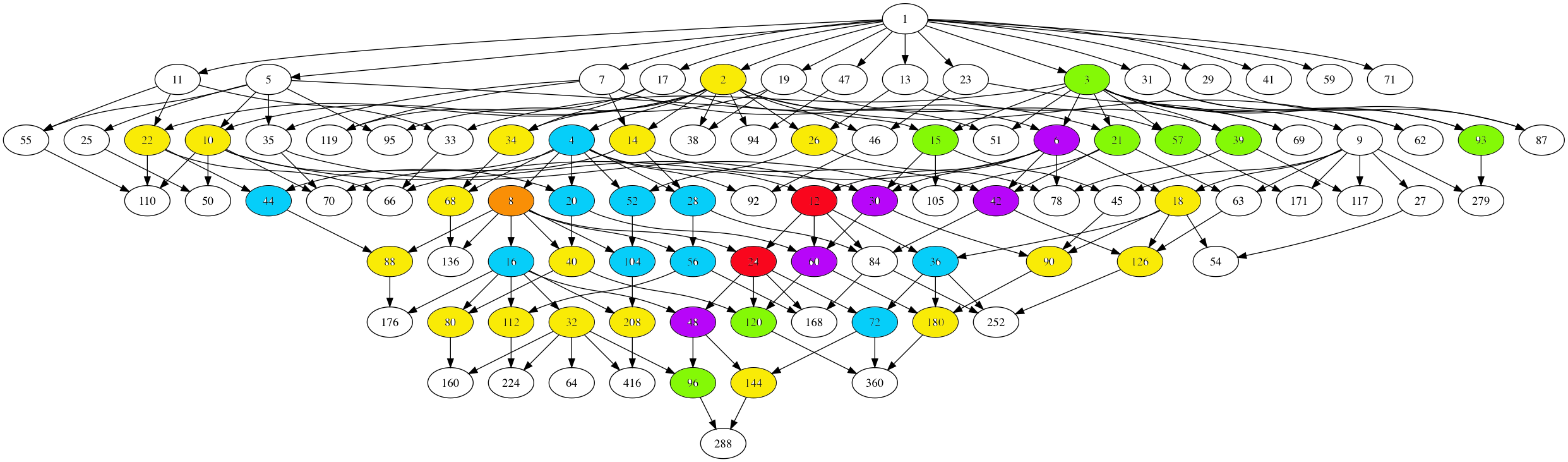The monstrous moonshine picture is the subgraph of Conway’s big picture consisting of all lattices needed to describe the 171 moonshine groups.

It consists of:

– exactly 218 vertices (that is, lattices), out of which

– 97 are number-lattices (that is of the form $M$ with $M$ a positive integer), and

– 121 are proper number-like lattices (that is of the form $M \frac{g}{h}$ with $M$ a positive integer, $h$ a divisor of $24$ and $1 \leq g \leq h$ with $(g,h)=1$).

The $97$ number lattices are closed under taking divisors, and the corresponding Hasse diagram has the following shapeHere, number-lattices have the same colour if they have the same local structure in the moonshine picture (that is, have a similar neighbourhood of proper number-like lattices).

There are 7 different types of local behaviour:

The white numbered lattices have no proper number-like neighbours in the picture.

The yellow number lattices (2,10,14,18,22,26,32,34,40,68,80,88,90,112,126,144,180,208 = 2M) have local structure

$\xymatrix{M \ar@{-}[r] & \color{yellow}{2M} \ar@{-}[r] & M \frac{1}{2}}$

which involves all $2$-nd (square) roots of unity centered at the lattice.

The green number lattices (3,15,21,39,57,93,96,120 = 3M) have local structure

$\xymatrix{& M \ar@[red]@{-}[d] & \\ M \frac{1}{3} \ar@[red]@{-}[r] & \color{green}{3M} \ar@[red]@{-}[r] & M \frac{2}{3}}$

which involve all $3$-rd roots of unity centered at the lattice.

The blue number lattices (4,16,20,28,36,44,52,56,72,104 = 4M) have as local structure

$\xymatrix{M \frac{1}{2} \ar@{-}[d] & & M \frac{1}{4} \ar@{-}[d] \\ 2M \ar@{-}[r] & \color{blue}{4M} \ar@{-}[r] & 2M \frac{1}{2} \ar@{-}[d] \\ M \ar@{-}[u] & & M \frac{3}{4}}$

and involve the $2$-nd and $4$-th root of unity centered at the lattice.

The purple number lattices (6,30,42,48,60 = 6M) have local structure

$\xymatrix{& M \frac{1}{3} \ar@[red]@{-}[d] & 2M \frac{1}{3} & M \frac{1}{6} \ar@[red]@{-}[d] & \\ M \ar@[red]@{-}[r] & 3M \ar@{-}[r] \ar@[red]@{-}[d] & \color{purple}{6M} \ar@{-}[r] \ar@[red]@{-}[u] \ar@[red]@{-}[d] & 3M \frac{1}{2} \ar@[red]@{-}[r] \ar@[red]@{-}[d] & M \frac{5}{6} \\ & M \frac{2}{3} & 2M \frac{2}{3} & M \frac{1}{2} & }$

and involve all $2$-nd, $3$-rd and $6$-th roots of unity centered at the lattice.

The unique brown number lattice 8 has local structure

$\xymatrix{& & 1 \frac{1}{4} \ar@{-}[d] & & 1 \frac{1}{8} \ar@{-}[d] & \\ & 1 \frac{1}{2} \ar@{-}[d] & 2 \frac{1}{2} \ar@{-}[r] \ar@{-}[d] & 1 \frac{3}{4} & 2 \frac{1}{4} \ar@{-}[r] & 1 \frac{5}{8} \\ 1 \ar@{-}[r] & 2 \ar@{-}[r] & 4 \ar@{-}[r] & \color{brown}{8} \ar@{-}[r] & 4 \frac{1}{2} \ar@{-}[d] \ar@{-}[u] & \\ & & & 1 \frac{7}{8} \ar@{-}[r] & 2 \frac{3}{4} \ar@{-}[r] & 1 \frac{3}{8}}$

which involves all $2$-nd, $4$-th and $8$-th roots of unity centered at $8$.

Finally, the local structure for the central red lattices $12,24 = 12M$ is

$\xymatrix{ M \frac{1}{12} \ar@[red]@{-}[dr] & M \frac{5}{12} \ar@[red]@{-}[d] & M \frac{3}{4} \ar@[red]@{-}[dl] & & M \frac{1}{6} \ar@[red]@{-}[dr] & M \frac{1}{2} \ar@[red]@{-}[d] & M \frac{5}{6} \ar@[red]@{-}[dl] \\ & 3M \frac{1}{4} \ar@{-}[dr] & 2M \frac{1}{6} \ar@[red]@{-}[d] & 4M \frac{1}{3} \ar@[red]@{-}[d] & 2M \frac{1}{3} \ar@[red]@{-}[d] & 3M \frac{1}{2} \ar@{-}[dl] & \\ & 2M \frac{1}{2} \ar@[red]@{-}[r] & 6M \frac{1}{2} \ar@{-}[dl] \ar@[red]@{-}[d] \ar@{-}[r] & \color{red}{12M} \ar@[red]@{-}[d] \ar@{-}[r] & 6M \ar@[red]@{-}[d] \ar@{-}[dr] \ar@[red]@{-}[r] & 2M & \\ & 3M \frac{3}{4} \ar@[red]@{-}[dl] \ar@[red]@{-}[d] \ar@[red]@{-}[dr] & 2M \frac{5}{6} & 4M \frac{2}{3} & 2M \frac{2}{3} & 3M \ar@[red]@{-}[dl] \ar@[red]@{-}[d] \ar@[red]@{-}[dr] & \\ M \frac{1}{4} & M \frac{7}{12} & M \frac{11}{12} & & M \frac{1}{3} & M \frac{2}{3} & M}$

It involves all $2$-nd, $3$-rd, $4$-th, $6$-th and $12$-th roots of unity with center $12M$.

No doubt this will be relevant in connecting moonshine with non-commutative geometry and issues of replicability as in Plazas’ paper Noncommutative Geometry of Groups like $\Gamma_0(N)$.

Another of my pet follow-up projects is to determine whether or not the monster group $\mathbb{M}$ dictates the shape of the moonshine picture.

That is, can one recover the 97 number lattices and their partition in 7 families starting from the set of element orders of $\mathbb{M}$, applying some set of simple rules?

One of these rules will follow from the two equivalent notations for lattices, and the two different sets of roots of unities centered at a given lattice. This will imply that if a number lattice belongs to a given family, certain divisors and multiples of it must belong to related families.

If this works out, it may be a first step towards a possibly new understanding of moonshine.

Published in groups math noncommutative